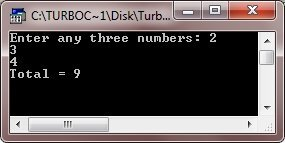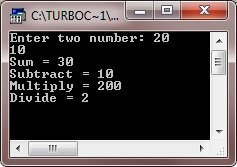# C++ Data Abstraction

Data abstraction is the process of representing the essential features without including the implementation details. In other words, you can say that, data abstraction refers to, providing only the essential features by hiding the background details.

To create abstraction, or to abstract data from outside the world, then follow these two steps to provide or hide the implementation details from outside the world:

• Members which are defined with a public label are accessible to the all parts of the program.
• And the members which are defined with a private label are not accessible to the code that uses the class.

## Data Abstraction Example Program in C++

Let's take some example program which illustrates data abstraction practically in C++. Here is the first example program demonstrating data abstraction in C++

```/* C++ Data Abstraction - Example Program */

#include<iostream.h>
#include<conio.h>
class ADD
{
public:
ADD(int i=0)
{
tot=i;
}
void addnumber(int num)
{
tot=tot+num;
}
int gettotal()
{
return tot;
}
private:
// hidden from outside the world
int tot;
};
void main()
{
clrscr();
ADD aob;
int a, b, c;
cout<<"Enter any three numbers: ";
cin>>a>>b>>c;
aob.addnumber(a);
aob.addnumber(b);
aob.addnumber(c);
cout<<"Total = "<<aob.gettotal();
getch();
}```

Here is the sample run of the above C++ program:Here is another example program, also demonstrating the data abstraction in C++

```/* C++ Data Abstraction - Example Program */

#include<iostream.h>
#include<conio.h>
class CALC
{
public:
CALC(int i=0)
{
res=i;
}
void addnumber(int num1, int num2)
{
res=num1+num2;
}
void subnumber(int num1, int num2)
{
res=num1-num2;
}
void mulnumber(int num1, int num2)
{
res=num1*num2;
}
void divnumber(int num1, int num2)
{
res=num1/num2;
}
int getresult()
{
return res;
}
private:
int res;
};
void main()
{
clrscr();
CALC cob;
int a, b;
cout<<"Enter two number: ";
cin>>a>>b;
cob.addnumber(a, b);
cout<<"Sum = "<<cob.getresult()<<"\n";
cob.subnumber(a, b);
cout<<"Subtract = "<<cob.getresult()<<"\n";
cob.mulnumber(a, b);
cout<<"Multiply = "<<cob.getresult()<<"\n";
cob.divnumber(a, b);
cout<<"Divide = "<<cob.getresult()<<"\n";
getch();
}```

Below is the sample run of the above C++ program:Tools
Calculator

Quick Links
Signup - Login - Give Online Test